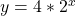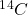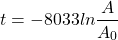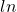# Chapter 9

## Unconstrained Growth Exercises

1. Let’s evaluate some exponential functions either in MATLAB or Python. For the expression:generate a set of values for y using values of x from -4 to 10 in increments of 1. Plot a graph of the relationship. Now try varying the constant and observe what happens to the graph. Do the same thing varying the exponential base. Finally, change the exponent to a negative number. How does each change impact the form and range of the graph?
2. Write a program to calculate the interest on an account where you initially save $100 per month over thirty years. Calculate the growth in the account assuming that you receive 1, 3, or 5 percent interest compounded annually. To keep the calculations fairly simple, only calculate the interest at the end of each year (having added$1200). Create a graph showing the growth of your savings over time for each of the three interest rates on the same figure. If you want to end up with a minimum of $100,000 in savings after 30 years, how much would you have to change the monthly savings amount to reach that amount at each interest rate? Alter your model to calculate that amount by substituting in different savings amounts in increments of$25 per month. Provide the codes for the original and new solutions along with a one page summary documenting your program and the results.
3. In 1946 Willard Libby and his colleagues were the first to recognize the existence of a radioactive isotope of carbon. The vast majority of carbon has the atomic weight 12 while radioactive carbon has the atomic weight of 14 (often represented as). This isotope is formed continually in the upper atmosphere by the interaction of neutrons produced by cosmic rays with nitrogen atoms and then becomes part of the carbon dioxide in the atmosphere (Bowman, 1990, p. 10). That carbon dioxide becomes mixed with the atmosphere and is absorbed by all living things. Once a plant or animal dies, it no longer participates in carbon exchange resulting in the radioactive decay of. Libby (1955) is credited with describing how to analyze theto determine the age of archeological and other objects. Although there are a number of assumptions concerning the historical levels ofin the atmosphere, its mixing and concentration across the world, and the potential for sample contamination, the simple model of radioactive decay ofcan be used to approximate the age of any object that was part of the biosphere. Using Libby’s estimated half-life of 5568 years, the radiocarbon age of an object is represented by the equation:where:
t = timeis the ratio of remainingatoms to the amount in the atmosphere
andis the natural logarithm
Write a program to create a graph of the number of atoms remaining in a sample versus time. What is the approximate age of a bone found with 0.77 percent of?

## Constrained Growth Exercises

1. To model constrained growth, you will build a population dynamics models for predator and prey which includes part of the interactions conceptualized under this topic. The interactions are based on a model formulated by an American demographer Alfred Lotka and an Italian physicist name Vito Volterra working independently (see a summary of this work at https://en.wikipedia.org/wiki/Lotka%E2%80%93Volterra_equations. The model expressed as a difference equation for rabbits and wolves is:

Rabbits(t+1) = Rabbits(t) +Rabbits(t) * Rabbit Growth Rate – Rabbits(t)*Rabbit Death Rate*Wolves(t)
Wolves(t+1)=Wolves (t) +Wolves(t)*Wolf Growth Rate*Rabbits(t)-Wolves(t)*Wolf Death Rate

Where:
t is the time increment

If we start with the basic growth model from interest and instead calculate rabbits, the only information we need is the rabbit growth and death rates and the initial number of wolves to satisfy the first equation. Similarly, we can use the basic growth rate model to model the wolf population needing only the wolf birth and death rates and the initial rabbit population. Create a model that incorporates these new factors and plot the population of rabbits and wolves on the same graph over time. Here are the parameters you should use to start. If your model works correctly, you should observe a cyclical pattern where the prey increases followed by an increase in predators and then a decline in both.

You should notice that the pattern that emerges is becoming increasingly unstable, with larger peaks and valleys. Change the model parameters to investigate how a more stable population might be achieved. Then discuss the design of a more complex model including the incorporation of additional components the data that would be needed to implement it. Discuss the findings of your model along with those concerning the design of the more complex model.

## Ball Toss Exercise

Using the information in chapter 9, program a simple model of a ball tossed straight up from a bridge. Model it as a falling object as discussed in section 9.3.1. Assume that the bridge is 15 meters above the ground and that it is thrown upward at a velocity of 25 m/s. Model its velocity and position over time until it reaches the ground. Discuss the questions below as part of your exercise.

1. What are the assumptions underlying the model?
2. What other functions would we need to add to the model to relax the major assumptions?